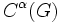# Poisson-Lie group

## Definition

A Poisson-Lie group$G$ is a differential manifold$G$ equipped with the following two additional structures:

• The structure of a Lie group
• The structure of a Poisson manifold (in other words, a Poisson algebra to the set$C^\alpha(G)$)

satisfying the following compatibility conditions: the group multiplication$G \times G \to G$ is a Poisson map from$G \times G$ with the Poisson product structure, to$G$.